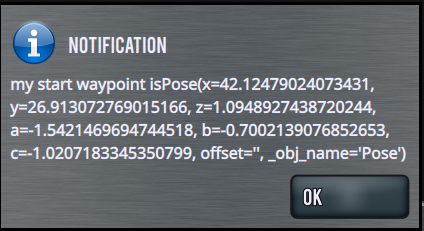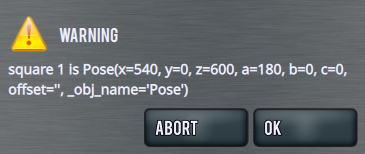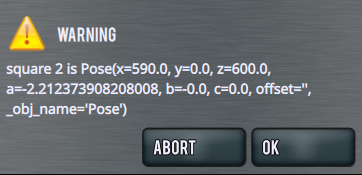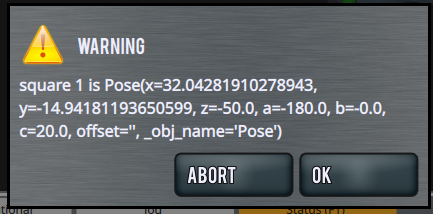# Trying to offset way points but having issues

Hey everyone. I am working on an example program with the robot moving checkers on a checker board to figure out how the waypoint offsets work. I am running into issues where I am not quite sure about the offset syntax and things are not working the way I would expect.

From the Documentation I didnt expect to need to create a new waypoint for my “offset points” but it appears that was needed. So I added three offset points in the header and just primed them with Zeros. I was expecting that when I set up the offsets it would pull any numbers not set by the offset from the original point. In this case “zero_square”

``````from robot_command.rpl import *

set_units("mm", "deg")
Perch = p[112.13211715221405, -91.20772033929825, 140.10269939899445, -177.47084809582782, -0.7044131289825072, -7.300005320292954, "checkerBox"]
zero_square = p[0.0005586846896221687, -0.0006692839633615222, -0.0003718444645528507, -177.47133557050287, -0.7002139076852653, -7.303903641714666, "checkerBox"]
start = p[0,0,0,0,0,0, "checkerBox"]
square_1 = p[0,0,0,0,0,0, "checkerBox"]
square_2 = p[0,0,0,0,0,0, "checkerBox"]

square = 5

# start = zero_square * Pose(z=50)
square_1 = zero_square * Pose(x=square/2, y= square/2, z=square)
square_2 = square_1 * Pose(x=square, y= square)

def main():
set_digital_out(1, False)

change_work_offset("checkerBox")
change_tool_offset("Gripper1")

start = zero_square * Pose(z=50)

notify("my start waypoint is" + str(start))
# movej(start)
# movel(start, v=0.25, a=0.3)
# sleep(2.0)
# movel(square_1, v=0.25, a=0.3)
# sleep(2.0)
# movel(square_2, v=0.25, a=0.3)
exit()

``````

From looking at confluence the `start = zero_square * Pose(z=50)` line should have set the start pose to be equal to “zero_square” but 50 on Z but when I run the program I get this for the value of the Pose:Any help would be appreciated.

Hi Joe!

I’m going to simplify your code a bit and I hope we can get to the bottom of things.

Have a look at this program:

``````from robot_command.rpl import *

set_units("mm", "deg")
square_1 = p[540, 0, 600, 180, 0, 0]

def main():
notify("square 1 is " + str(square_1), warning=True)
square_2 = square_1 * Pose(x=50)
notify("square 2 is " + str(square_2), warning=True)
movej(square_2)
pass

``````

And here’s what the pop ups tell us about `square_1` and `square_2`:`square_1` comes out as we expect.
`square_2` is offset by 50mm in x, as we would expect, but there’s something going on with the A axis value that I don’t understand. @machinekoder might have some insight.

Note that square_2 does not have to be created above the main function for this code to run. You can create waypoints ‘on the fly’ in your program, and the robot will move to them successfully.

I haven’t repeated your example with a user frame active, but I don’t think there will be a difference.

1 Like

Here’s an example of creating a grid of 50mm spaced waypoints for a checkers game. The example assumes that the lower left corner of your grid is [0,0,0,0,0,0], so you will need to create a user frame (work offset) to zero out that position on your physical board.

``````from robot_command.rpl import *

set_units("mm", "deg")
square_1 = p[0, 0, 0, 0, 0, 0]

WIDTH = 800
ROWS = 8
COLUMNS = ROWS
SQUARE_SIZE = 50

# assume origin is center of the lower left square, 8x8 board
grid = []

for i in range(ROWS):
# create a new row
grid.append([])
for j in range(COLUMNS):
x_offset = i*SQUARE_SIZE
y_offset = j*SQUARE_SIZE
grid[i].append(square_1 * Pose(x=x_offset, y=y_offset))

def main():
notify("square 1 is " + str(square_1), warning=True)
notify("4th row, 2nd column is " + str(grid), warning=True)

for i in range(ROWS):
for j in range(COLUMNS):
movej(grid[i][j])
sleep(.5)
pass

``````

Video:

1 Like

Poses have no notion about their units. So to apply an offset for anything that contains rotations, you need to convert it to ROS units at the moment.

``````units = get_units()
new_pose = (pose .to_ros_units(*units) * Pose(x=10).to_ros_units(*units)).from_ros_units(*units)
``````

Yes, I know it’s very inconvenient at the moment. If you want to simplify things for now. Please use Radians as angular unit type.

Unit capture for the poses is something we are working on.

Thanks for the feedback. Having the syntax broke out for me is helpful. I’ll try this!

@machinekoder I am still having issues with odd math when I use variables in place of hard numbers in the offsets.

The code below should offset X, Y by 25 and z by 50. It comes out x=32.xxxx and y=–14.xxxx and z=50.000001

``````from robot_command.rpl import *

set_units("mm", "deg")
units = get_units()
zero_square = p[0,0,0,-180, 0, 20, "checkerBox"]
# square_1 = p[0,0,0,0,0,0, "checkerBox"]
# square_2 = p[0,0,0,0,0,0, "checkerBox"]

square = float(50)

def main():
set_digital_out(1, False)

change_work_offset("checkerBox")
# change_tool_offset("Gripper1")

square_1 = (zero_square.to_ros_units(*units) * Pose(x=square/2, y=square/2, z=square).to_ros_units(
*units)).from_ros_units(*units)
square_2 = (zero_square.to_ros_units(*units) * Pose(x=square/2, y=square/2, z=square).to_ros_units(
*units)).from_ros_units(*units)

notify("square 1 is " + str(square_1), warning=True)
notify("square 2 is " + str(square_2), warning=True)
exit()
``````Setting the initial position with radians and with hard numbers gives similar results. Im not sure why units matter in this case. I am adding 50 to the existing value…

``````from robot_command.rpl import *
zero_square = p[0, 0, 0, -3.140044502568047, -0.001657591551521115, 0.3499429675459741, "checkerBox"]
units = get_units()
# square_1 = p[0,0,0,0,0,0, "checkerBox"]
# square_2 = p[0,0,0,0,0,0, "checkerBox"]

square = float(50)

def main():
set_digital_out(1, False)
change_work_offset("checkerBox")

start = zero_square * Pose(z=50)
square_1 = zero_square * Pose(x=square / 2, y=square / 2, z=square)
square_2 = zero_square * Pose(x=square / 2, y=square / 2, z=square)
square_3 = zero_square * Pose(x=25, y=25, z=50)
square_4 = zero_square * Pose(x=50, y=50, z=50)

notify("start is " + str(start), warning=True)
notify("square 1 is " + str(square_1), warning=True)
notify("square 2 is " + str(square_2), warning=True)
notify("square 3 is " + str(square_3), warning=True)
notify("square 4 is " + str(square_4), warning=True)
exit()

``````

its also changing the zeros in the “start” that should only be offset in ZFollow up on this. After discussing more offline, Alex suggested I invert the offset command like this

``````square_1 =Pose(x=square / 2, y=square / 2, z=square) *  zero_square
``````

and check the results.

That, combined with changing to radians is now giving me the results I was hoping for.

The code below works as expected and give me the start of what I am after.

``````from robot_command.rpl import *
zero_point = p[0, 0, 0, -3.140044502568047, 0, 0, "checkerBox"]
zero_square = p[25, 25, 0, -3.140044502568047, 0, 0, "checkerBox"]
units = get_units()
# square_1 = p[0,0,0,0,0,0, "checkerBox"]
# square_2 = p[0,0,0,0,0,0, "checkerBox"]

square = float(50)

def main():
set_digital_out(1, False)
change_work_offset("checkerBox")
change_tool_offset("Gripper1")

start = Pose(z=50) * zero_point
square_1 = Pose(z=square) * zero_square
square_2 = Pose(x=square, z=square) * zero_square
square_3 = Pose(x=square*2, z=50) * zero_square
square_4 = Pose(x=square*3, z=50) * zero_square

# notify("start is " + str(start), warning=True)
movel(start, a=0.4, v=0.2)
sleep(2.0)
# notify("square 1 is " + str(square_1), warning=True)
movel(square_1, a=0.4, v=0.2)
sleep(2.0)
# notify("square 2 is " + str(square_2), warning=True)
movel(square_2, a=0.4, v=0.2)
sleep(2.0)
# notify("square 3 is " + str(square_3), warning=True)
movel(square_3, a=0.4, v=0.2)
sleep(2.0)
# notify("square 4 is " + str(square_4), warning=True)
movel(square_4, a=0.4, v=0.2)
sleep(2.0)
exit()
``````
1 Like